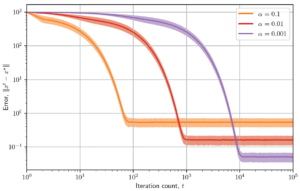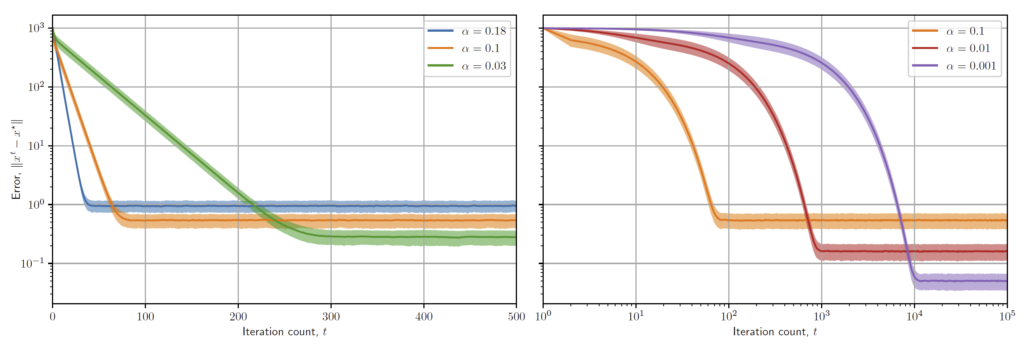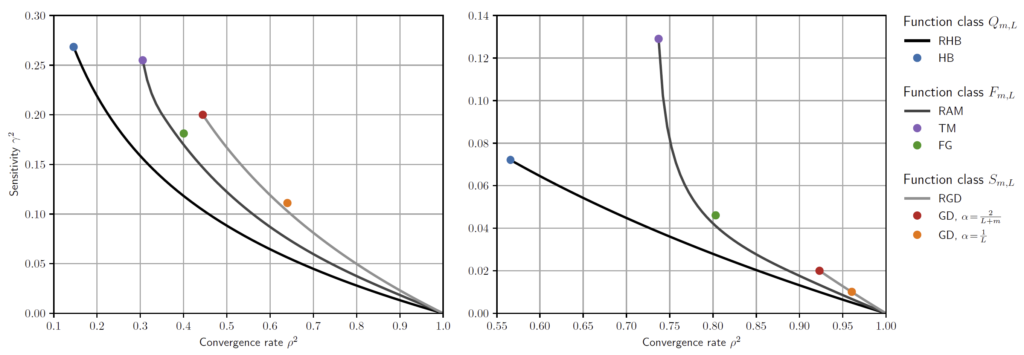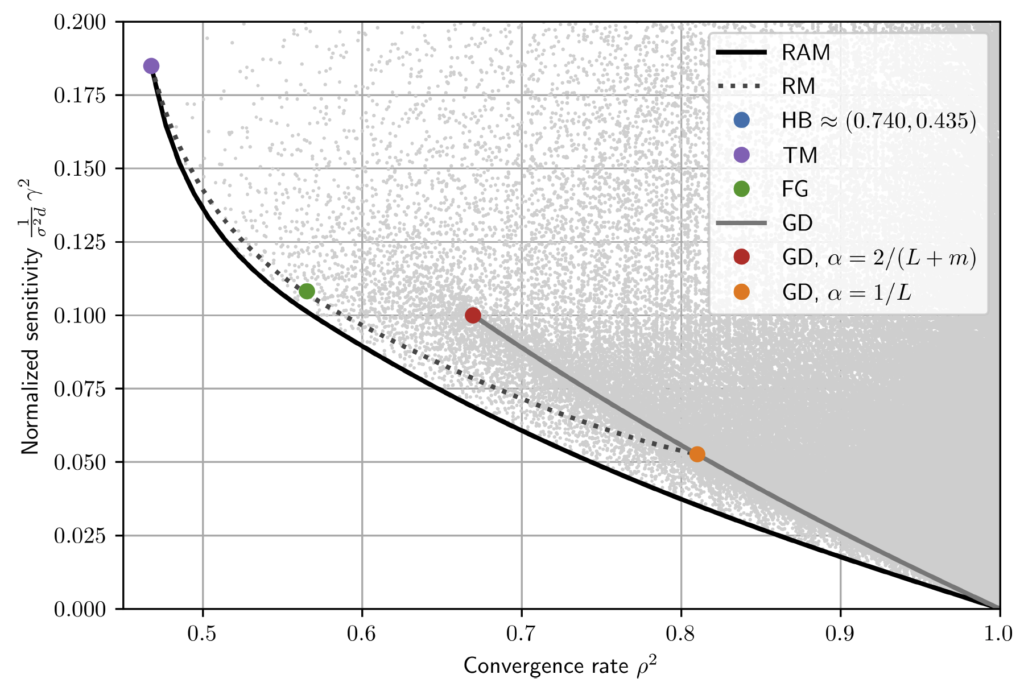# Speed-robustness trade-off for optimization algorithms

Excited to share a new manuscript, by Bryan Van Scoy and me titled “The Speed-Robustness Trade-Off for First-Order Methods with Additive Gradient Noise”. It is now available on arXiv. I also have some slides here.

## BackgroundWhen solving an optimization problem, a ubiquitous approach is to use an iterative algorithm that finds progressively better candidate solutions until a satisfactory solution is obtained. In cases where the problem instances are very large, such as in machine learning applications, gradient-based iterative methods are the most popular. Think of the task of finding the point of highest elevation in a vast landscape. At each step, you measure the slope of the terrain, and move in the direction of steepest increase. Since we don’t know what the terrain shape will be ahead of time, we want algorithms that work well for a wide variety of terrains. For this reason, a popular way of evaluating algorithms is to look at their worst-case convergence rate on a given class of functions. For example, we might be solving a large linear regression problem, so we know ahead of time that the function we are optimizing is a convex quadratic. Much work has gone into finding algorithms that are “optimal” for a given class of functions. For example, we know that the Heavy Ball method is optimal for convex quadratics and the Triple Momentum Method is optimal for smooth strongly convex functions.

However, worst-case convergence rate is not always the best metric to use. To continue the hill-climbing analogy, the terrain might be covered with grass and rocks, so the local slope measurements we take might be misleading. In such cases, we might want to design our algorithm differently, perhaps including some form of averaging to mitigate this uncertainty in our measurements, or taking smaller steps so we don’t move too far in the wrong direction. In the context of machine learning, evaluating the gradient of the function we want to optimize might be a costly procedure that can only be done approximately. In other words, our gradients are noisy. It turns out that algorithms designed to perform “optimally” in the worst-case sense don’t usually fare well when used in noisy scenarios.

A more subtle point is that not all metrics used to quantify performance are suitable for algorithm design. For example, when the class of functions of interest is very large (everything from a desert plain to rocky canyons), it is difficult to design an algorithm that performs well in the worst case without being excessively conservative. For example, we expect some terrains to be more challenging than others, but if we try to design a single algorithm whose worst-case performance is as good as possible, it typically leads to algorithms that always perform as their worst case. Even when presented with “easy” terrains, such algorithms perform as if the terrain was more difficult. In summary, algorithms that perform well in the worst case don’t necessarily perform well on average. Another analogy: if you’re constantly paranoid about missing flights so you always arrive at the airport 5 hours early, then you will never miss a flight. But your trips will take longer than they should. Is it worth it? This depends on your sensitivity to risk!

## ContributionsAlthough Gradient Descent makes this trade-off explicit, similar trade-offs exist for all other algorithms. The problem is that for Heavy Ball or Triple Momentum, for example, these algorithms have 2 or 3 tunable parameters; it’s not clear how they should be tuned to get the best balance between speed and sensitivity. It’s not even clear that these are good starting points at all! What if an entirely different algorithm performed better? In our paper, we investigated three function classes:

• $Q_{m,L}$: Strongly convex quadratic functions whose Hessian has eigenvalues in the range ($m$,$L$).
• $F_{m,L}$: Smooth strongly convex functions whose strong convexity and Lipschitz parameters are $m$ and $L$, respectively.
• $S_{m,L}$: Functions that satisfy the smooth and strongly convex criterion with ($m$, $L$) with respect to the optimal point, but not necessarily all pairs of points. Such functions are not necessarily convex, but always have a unique optimal point.
These function classes are nested: $Q_{m,L} \subseteq F_{m,L} \subseteq S_{m,L}$. Therefore, we expect algorithms designed for $Q_{m,L}$ to be both faster and less sensitive than those designed for $F_{m,L}$ or $S_{m,L}$, since it is a smaller class of functions. In our work, we used different techniques to address each function class, but a common thread was that we searched for Lyapunov functions to certify robust stability.

For each function class, we designed a family of algorithms parameterized by a single scalar tuning parameter. This parameter explicitly trades off our the worst-case convergence rate and the sensitivity to noise. We named our designs RHB, RAM, and RGD, respectively. Like the Gradient Descent example, our algorithm designs can be easily tuned depending on the desired balance. As a side note, Gradient Descent is not optimal for any of the three function classes mentioned above! Our designs are structurally similar to Triple Momentum, but with different tunings. When tuned in the traditional way (fastest worst-case convergence rate possible), we actually recover the best-known methods from the literature. We can also produce nice trade-off curves that show how each algorithm family performs. In the left panel, we used $m=1$ and $L=5$. In the right panel, we used $m=1$ and $L=50$.The challenge was to produce algorithms that achieved a near-optimal trade-off, but also had simple algebraic forms that could be easily implemented on a computer. Our designs are not actually Pareto-optimal, but they are very close, and strike a good balance between optimality and simplicity. Our designs have the parametric form: $x^{t+1} = x^t-\alpha\, \nabla\! f\bigl( x^t + \eta\,(x^t-x^{t-1})\bigr) + \beta\,(x^t-x^{t-1})$For example, our RAM method, which is near-optimal for the class $F_{m,L}$, is given by: $\alpha = \frac{(1+\rho)(1-\rho)^2}{m}, \quad \beta = \rho\,\frac{L\,(1-\rho+2\rho^2)-m\,(1+\rho)}{(L-m)(3-\rho)}, \quad\text{and}\quad \eta = \rho\,\frac{L\,(1-\rho^2)-m\,(1+2\rho-\rho^2)}{(L-m)(3-\rho)(1-\rho^2)}$Here, $1-\sqrt{\frac{m}{L}} \leq \rho \lt 1$ is the tuning parameter that controls the worst-case contraction factor. Smaller $\rho$ means faster worst-case convergence. When chosen as small as possible, we recover the Triple Momentum Method.

To verify near-optimality of our designs, we performed brute-force searches and produced plots such as the one below, which is for $F_{m,L}$ with $m=1$ and $L=10$. We searched through all 3-parameter algorithms with Triple-Momentum structure, each producing a single gray dot. We also included other popular algorithms such as Heavy Ball (HB), Triple Momentum (TM), Nesterov’s method (FG), and Gradient Descent (GD). Our algorithm (RAM), shows up as a curve rather than a dot because it is a one-parameter tunable family. As we can see, none of the gray dots lie below the RAM curve. We also plotted the Robust Momentum Method (RM), which was an earlier attempt at making this sort of trade-off that we presented at ACC’18 in Milwaukee.There is a great deal more, but it’s too much to include in this blog post. If you find this interesting, take a look at our preprint! For comments/discussion, I posted a link on Twitter.